# Analysis of Truss with ExamplesReading time: 1 minute

Learn truss analysis methods with examples. Analysis of trusses by the methods of joints and by the methods of section is explained in the article. We know the basics of equilibrium of bodies; we will now discuss the trusses that are used in making stable load-bearing structures. The examples of these are the sides of the bridges or tall TV towers or towers that carry electricity wires. Schematic diagram of a structure on the side of a bridge is drawn in figure 1.The structure shown in figure 1 is essentially a two-dimensional structure. This is known as a plane truss. On the other hand, a microwave or mobile phone tower is a three-dimensional structure. Thus there are two categories of trusses - Plane trusses like on the sides of a bridge and space trusses like the TV towers. In this course, we will be concentrating on plane trusses in which the basis elements are stuck together in a plane. To motivate the structure of a plane truss, let me take a slender rod (12) between points 1 and 2 and attach it to a fixed pin joint at 1 (see figure 2).Now I put a pin (pin2) at point 2 at the upper end and hang a weight W on it. The question is if we want to hold the weight at that point, what other minimum supports should we provide? For rods we are to make only pin joints (We assume everything is in this plane and the structures does not topple side ways). Since rod (12) tends to turn clockwise, we stop the rightward movement of point 2 by connecting a rod (23) on it and then stop point 3 from moving to the right by connecting it to point 1 by another rod (13). All the joints in this structure are pin joints. However, despite all this the entire structure still has a tendency to turn to turn clockwise because there is a torque on it due to W. To counter this, we attach a wheel on point 3 and put it on the ground. This is the bare minimum that we require to hold the weight is place. The triangle made by rods forms the basis of a plane truss. Note: One may ask at this point as to why as we need the horizontal rod (13). It is because point 3 will otherwise keep moving to the right making the whole structure unstable. Rod (13) has two forces acting on it: one vertical force due to the wheel and the other at end 2. However these two forces cannot be collinear so without the rod (13) the system will not be in equilibrium. Generally, in a truss each joint must be connected to at least three rods or two rods and one external support. Let us now analyze forces in the structure that just formed. For simplicity I take the lengths of all rods to be equal. To get the forces I look at all the forces on each pin and find conditions under which the pins are in equilibrium. The first thing we note that each rod in equilibrium under the influence of two forces applied by the pins at their ends. As I discussed in the previous lecture, in this situation the forces have to be collinear and therefore along the rods only. Thus each rod is under a tensile or compressive force. Thus rods (12), (23) and (13) experience forces as shown in figure 3.Notice that we have taken all the forces to be compressive. If the actual forces are tensile, the answer will come out to be negative. Let us now look at pin 2. The only forces acting on pin 2 are F12 due to rod (12) and F23 due to rod (23). Further, it is pulled down by the weight W. Thus forces acting on pin 2 look like shown in figure 4.Applying equilibrium condition to pin (2) givesLet us now look at pin 3 (see figure 4). It is in equilibrium under forces F23, normal reaction N and a horizontal force F13. Applying equilibrium condition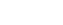givesSince the direction of F13 is coming out to be negative, the direction should be opposite to that assumed. Balance of forces in the vertical direction gives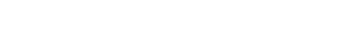Thus we see that the weight is held with these three rods. The structure is determinate and it holds the weight in place. Even if we replace the pin joints by a small plate (known as gusset plate) with two or three pins in these, the analysis remains pretty much the same because the pins are so close together that they hardly create any moment about the joints. Even if the rods are welded together at the joints, to a great degree of accuracy most of the force is carried longitudinally on the rods, although some very small (negligible) moment is created by the joints and may be by possible bending of the rods. Now we are ready to build a truss and analyze it. We are going to build it by adding more and more of triangles together. As you can see, when we add these triangles, the member of joints j and the number of members (rods) m are related as follows:

m = 2j - 3

This makes a truss statically determinate. This is easily understood as follows. First consider the entire truss as one system. If it is to be statically determinate, there should be only three unknown forces on it because for forces in a plane there are three equilibrium conditions. Fixing one of its ends a pin joint and putting the other one on a roller does that (roller also gives the additional advantage that it can help in adjusting any change in the length of a member due to deformations). If we wish to determine these external forces and the force in each member of the truss, the total number of unknowns becomes m + 3. We solve for these unknowns by writing equilibrium conditions for each pin; there will be 2j such equations. For the system to be determinate we should have m + 3 = 2j , which is the condition given above. If we add any more members, these are redundant. On the other hand, less number of members will make the truss unstable and it will collapse when loaded. This will happen because the truss will not be able to provide the required number of forces for all equilibrium conditions to be satisfied. Statically determinate trusses are known as simple trusses. Exercise 1: Shown in figure 5 are three commonly used trusses on the sides of bridges. Show that all three of them are simple trusses.You may ask why we put trusses on bridges. As our later analysis will show they distribute the load over all elements and thereby making the bridge stronger. We now wish to obtain the forces generated in various arms of a truss when it is loaded externally. This is done under the following assumptions:
1. If the middle line of the members of a truss meet at a point that point is taken as a pin joint. This is a very god assumption because as we have seen earlier while introducing a truss (triangle with pin joint), the load is transferred on to other member of the trusses so that forces remain essentially collinear with the member.
2. All external loads are applied on pin connections.
3. All members' weight is equally divided on connecting pins.
There are two methods of determining forces in the members of a truss - Method of joints and method of sections. We start with the method of joints:

## Truss Analysis - Method of joints:

In method of joints, we look at the equilibrium of the pin at the joints. Since the forces are concurrent at the pin, there is no moment equation and only two equations for equilibrium viz.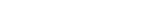. Therefore we start our analysis at a point where one known load and at most two unknown forces are there. The weight of each member is divided into two halves and that is supported by each pin. To an extent, we have already alluded to this method while introducing trusses. Let us illustrate it by two examples. Example 1: As the first example, I take truss ABCDEF as shown in figure 6 and load it at point E by 5000N. The length of small members of the truss is 4m and that of the diagonal members is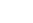m. I will now find the forces in each member of this truss assuming them to be weightless.We take each point to be a pin joint and start balancing forces on each of the pins. Since pin E has an external load of 5000N one may want to start from there. However, E point has more than 2 unknown forces so we cannot start at E. We therefore first treat the truss as a whole and find reactions of ground at points A and D because then at points A and D their will remain only two unknown forces. The horizontal reaction Nx at point A is zero because there is no external horizontal force on the system. To find N2 I take moment about A to get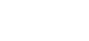which through equation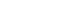gives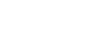In method of joints, let us now start at pin A and balance the various forces. We already anticipate the direction and show their approximately at A (figure 7). All the angles that the diagonals make are 45° .The only equations we now have worry about are the force balance equations.Keep in mind that the force on the member AB and AF going to be opposite to the forces on the pin ( Newton 's IIIrd law). Therefore force on member AB is compressive (pushes pin A away) whereas that on AF is tensile (pulls A towards itself). Next I consider joint F where force AF is known and two forces BF and FE are unknown. For pint FNext I go to point B since now there are only two unknown forces there. At point B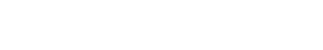Negative sign shows that whereas we have shown FBE to be compressive, it is actually tensile.Next I consider point C and balance the forces there. I have already anticipated the direction of the forces and shown FCE to be tensile whereas FCD to be compressiveNext I go to pin D where the normal reaction is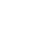N and balance forces there.Thus forces in various members of the truss have been determined. They areYou may be wondering how we got all the forces without using equations at all joints. Recall that is how we had obtained the statical determinacy condition. We did not have to use all joints because already we had treated the system as a whole and had gotten two equations from there. So one joint - in this case E - does not have to be analyzed. However, given that the truss is statically determinate, all these forces must balance at point E, where the load has been applied, also. I will leave this as an exercise for you. Next I ask how the situation would change if each member of the truss had weight. Suppose each members weighs 500N, then assuming that the load is divided equally between two pins holding the member the loading of the truss would appear as given in figure 8 (loading due to the weight as shown in red). Except at points A and D the loading due to the weight is 750N; at the A and D points it is 500N.Now the external reaction at each end will be.The extra 2000N can be calculated either from the moment equation or straightaway by realizing that the new added weight is perfectly symmetric about the centre of the truss and therefore will be equally divided between the two supports. For balancing forces at other pins, we follow the same procedure as above, keeping in mind though that each pin now has an external loading due to the weight of each member. I'll solve for forces in some member of the truss. Looking at pin A, we getNext we move to point F and see that the forces areOne can similarly solve for other pins in the truss and I leave that as an exercise for you. Having demonstrated to you the method of joints, we now move on to see the method of sections that directly gives the force on a desired member of the truss.

## Truss Analysis - Method of sections:

As the name suggests in method of sections we make sections through a truss and then calculate the force in the members of the truss though which the cut is made. For example, if I take the problem we just solved in the method of joints and make a section S1, S2 (see figure 9), we will be able to determine the forces in members BC, BE and FE by considering the equilibrium of the portion to the left or the right of the section.Let me now illustrate this. As in the method of joints, we start by first determining the reactions at the external support of the truss by considering it as a whole rigid body. In the present particular case, this gives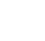N at D and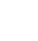N at A. Now let us consider the section of the truss on the left (see figure 10).Since this entire section is in equilibrium,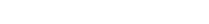. Notice that we are now using all three equations for equilibrium since the forces in individual members are not concurrent. The direction of force in each member, one can pretty much guess by inspection. Thus the force in the section of members BE must be pointing down because there is no other member that can give a downward force to counterbalanceN reaction at A. This clearly tells us that F BE is tensile. Similarly, to counter the torque about B generated byN force at A, the force on FE should also be from F to E. Thus this force is also tensile. If we next consider the balance of torque about A,N and FFE do not give any torque about A. So to counter torque generated by FBE , the force on BC must act towards B, thereby making the force compressive. Let us now calculate individual forces. FFE is easiest to calculate. For this we take the moment about B. This gives 4 ×= 4 × F FE F FE =N Next we calculate FBE . For this, we use the equation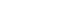. It gives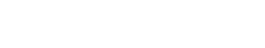Finally to calculate FBC , we can use either the equation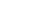about A or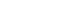Thus we have determined forces in these three members directly without calculating forces going from one joint to another joint and have saved a lot of time and effort in the process. The forces on the right section will be opposite to those on the left sections at points through which the section is cut. This can be used to check our answer, and I leave it as an exercise for you. After this illustration let me put down the steps that are taken to solve for forces in members of a truss by method of sections: 1. Make a cut to divide the truss into section, passing the cut through members where the force is needed. 2. Make the cut through three member of a truss because with three equilibrium equations viz.we can solve for a maximum of three forces. 3. Apply equilibrium conditions and solve for the desired forces. In applying method of sections, ingenuity lies in making a proper. The method after a way of directly calculating desired force circumventing the hard work involved in applying the method of joints where one must solve for each joint.